This book is archived and will be removed July 6, 2022. Please use the updated version.

Capital Budgeting Decisions

# 66 Use Discounted Cash Flow Models to Make Capital Investment Decisions

Your company, Rudolph Incorporated, has begun analyzing two potential future project alternatives that have passed the basic screening using the non–time value methods of determining the payback period and the accounting rate of return. Both proposed projects seem reasonable, but your company typically selects only one option to pursue. Which one should you choose? How will you decide? A discounted cash flow model can assist with this process. In this section, we will discuss two commonly used time value of money–based options: the net present value method (NPV) and the internal rate of return (IRR). Both of these methods are based on the discounted cash flow process.

### Fundamentals of the Discounted Cash Flow Model

The discount cash flow model assigns a value to a business opportunity using time-value measurement tools. The model considers future cash flows of the project, discounts them back to present time, and compares the outcome to an expected rate of return. If the outcome exceeds the expected rate of return and initial investment cost, the company would consider the investment. If the outcome does not exceed the expected rate of return or the initial investment, the company may not consider investment. When considering the discounted cash flow process, the time value of money plays a major role.

### Time Value-Based Methods

As previously discussed, time value of money methods assume that the value of money today is worth more now than in the future. The payback period and accounting rate of return methods do not consider this concept when performing calculations and analyzing results. That is why they are typically only used as basic screening tools. To decide the best option between alternatives, a company performs preference measurement using tools, such as net present value and internal rate of return that do consider the time value of money concept. Net present value (NPV) discounts future cash flows to their present value at the expected rate of return and compares that to the initial investment. NPV does not determine the actual rate of return earned by a project. The internal rate of return (IRR) shows the profitability or growth potential of an investment at the point where NPV equals zero, so it determines the actual rate of return a project earns. As the name implies, net present value is stated in dollars, whereas the internal rate of return is stated as an interest rate. Both NPV and IRR require the company to determine a rate of return to be used as the target return rate, such as the minimum required rate of return or the weighted average cost of capital, which will be discussed in Balanced Scorecard and Other Performance Measures.

A positive NPV implies that the present value of the cash inflows from the project are greater than the present value of the cash outflows, which represent the expenses and costs associated with the project. In an NPV calculation, a positive NPV is typically considered a potentially good investment or project. However, other extenuating circumstances should be considered. For example, the company might not wish to borrow the necessary funding to make the investment because the company might be anticipating a downturn in the national economy.

An IRR analysis compares the calculated IRR with either a predetermined rate of return or the cost of borrowing the money to invest in the project in order to determine whether a potential investment or project is favorable. For example, assume that the investment or equipment purchase is expected to generate an IRR of 15% and the company’s expected rate of return is 12%. In this case, similar to the NPV calculation, we assume that the proposed investment would be undertaken. However, remember that other factors must be considered, as they are with NPV.

When considering cash inflows—whether using NPV or IRR—the accountant should examine both profits generated or expenses reduced. Investments that are made may generate additional revenue or could reduce production costs. Both cases assume that the new product or other type of investment generates a positive cash inflow that will be compared to the cost outflows to determine whether there is an overall positive or negative net present value.

Additionally, a company would determine whether the projects being considered are mutually exclusive or not. If the projects or investment options are mutually exclusive, the company can evaluate and identify more than one alternative as a viable project or investment, but they can only invest in one option. For example, if a company needs one new delivery truck, it might solicit proposals from five different truck dealers and conduct NPV and IRR evaluations. Even if all proposals pass the financial requirements of the NPV and IRR methods, only one proposal will be accepted.

Another consideration occurs when a company has the ability to evaluate and accept multiple proposals. For example, an automobile manufacturer is considering expanding its number of dealerships in the United States over the next ten-year period and has allocated ?30,000,000 to buy the land. They could purchase any number of properties. They conduct NPV and IRR analyses of fifteen properties and determine that four meet their required standards and market feasibility needs and then purchase those four properties. The opportunities were not mutually exclusive: the number of properties purchased was driven by research and expansion projections, not by their need for only one option.

Capital Budgeting Decisions

Gearhead Outfitters has expanded to many locations throughout its twenty-plus years in business. How did company management decide to expand? One of the financial tools a business can use is capital budgeting, which addresses many different issues involving the use of current cash flow for future return. As you’ve learned, capital outlay decisions can be evaluated through payback period, net present value, and methods involving rates of return.

With this in mind, think about the capital budgeting issues Gearhead’s management might have faced. For example, in deciding to expand, should the company buy a building or lease one? What method should be used to evaluate this? Purchasing a building might require more initial outlay, but the company will retain an asset. How will such a decision affect the bottom line? With respect to equipment, Gearhead could maintain a fleet of vehicles. Should the vehicles be purchased or leased? What will need to be considered in the process?

In developing and maintaining its strategy for sustainability, a business must not only consider day-to-day operations, but also address long-term decisions. Common capital budgeting items like equipment purchases to increase efficiency or reduce costs, decisions about replacement versus repair, and expansion all involve significant cash outlay. How will these items be evaluated? How long will recouping the initial investment take? How much revenue will be generated (or costs saved) through capital outlay? Does the company require a minimum rate of return before it moves forward with investment? If so, how is that return determined? Considering Gearhead’s decision to expand, what are some specific capital budgeting decisions important for the company to consider in their long-term strategy?

#### Basic Characteristics of the Net Present Value Model

Net present value helps companies choose between alternatives at a particular point in time by determining which produces the higher NPV. To determine the NPV, the initial investment is subtracted from the present value of cash inflows and outflows associated with a project at a required rate of return. If the outcome is positive, the company should consider investment. If the outcome is negative, the company would forgo investment.

We previously discussed the calculation for present value using the present value tables, where n is the number of years and i is the expected interest rate. Once the present value factor is determined, it is multiplied by the expected net cash flows to produce the present value of future cash flows. The initial investment is subtracted from this present value calculation to determine the net present value.Recall that the Present Value of ?1 table is used for a lump sum payout, whereas the Present Value of an Ordinary Annuity table is used for a series of equal payments occurring at the end of each period. Taking this distinction one step further, NPV requires use of different tables depending on whether the future cash flows are equal or unequal in each time period. If the cash flows each period are equal, the company uses the Present Value of an Ordinary Annuity table, where the present value factor is multiplied by the cash flow amount for one period to get the present value. If the cash flows each period are unequal, the company uses the Present Value of ?1 table, where the total present value is the sum of each of the unequal cash flows multiplied by the appropriate present value factor for each time period. This concept is discussed in the following example.

Assume that your company, Rudolph Incorporated, is determining the NPV for a new X-ray machine. The X-ray machine has an initial investment of ?200,000 and an expected cash flow of ?40,000 each period for the next 10 years. The expected ?40,000 cash flows from the new X-ray machine can be attributed to either additional revenue generated or cost savings realized by more efficient operations of the new machine. Since these annual cash flows of ?40,000 are the same amount in each period over the ten-years this will be a stream of annuity amounts received. The required rate of return on such an investment is 8%. The present value factor (i = 8, n = 10) is 6.710 using the Present Value of an Ordinary Annuity table. Multiplying the present value factor (6.710) by the equal cash flow (?40,000) gives a present value of ?268,400. NPV is found by taking the present value of ?268,400 and subtracting the initial investment of ?200,000 to arrive at ?68,400. This is a positive NPV, so the company would consider investment.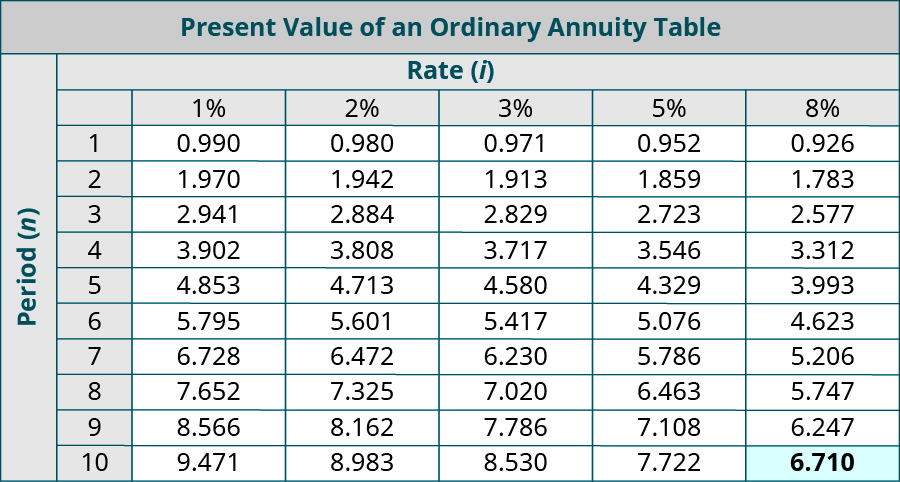If there are two investments that have a positive NPV, and the investments are mutually exclusive, meaning only one can be chosen, the more profitable of the two investments is typically the appropriate one for a company to choose. We can also use the profitability index to compare them. The profitability index measures the amount of profit returned for each dollar invested in a project. This is particularly useful when projects being evaluated are of a different size, as the profitability index scales the projects to make them comparable. The profitability index is found by taking the present value of the net cash flows and dividing by the initial investment cost.For example, Rudolph Incorporated is considering the X-ray machine that had present value cash flows of ?268,400 (not considering salvage value) and an initial investment cost of ?200,000. Another X-ray equipment option, option B, produces present value cash flows of ?290,000 and an initial investment cost of ?240,000. The profitability index is computed as follows.

$$\begin{array}{}\\ \\ \text{Option A:}\phantom{\rule{0.2em}{0ex}}\frac{?268,400}{?200,000}=1.342\hfill \\ \text{Option B:}\phantom{\rule{0.2em}{0ex}}\frac{?290,000}{?240,000}=1.208\hfill \end{array}$$

Based on this outcome, the company would invest in Option A, the project with a higher profitability index of 1.342.

If there were unequal cash flows each period, the Present Value of ?1 table would be used with a more complex calculation. Each year’s present value factor is determined and multiplied by that year’s cash flow. Then all cash flows are added together to get one overall present value figure. This overall present value figure is used when finding the difference between present value and the initial investment cost.

For example, let’s say the X-ray machine information is the same, except now cash flows are as follows: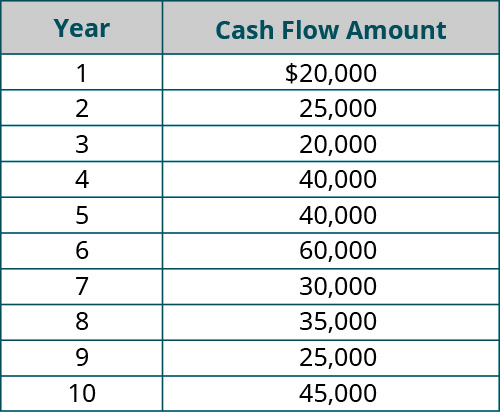To find the overall present value, the following calculations take place using the present value of ?1 table.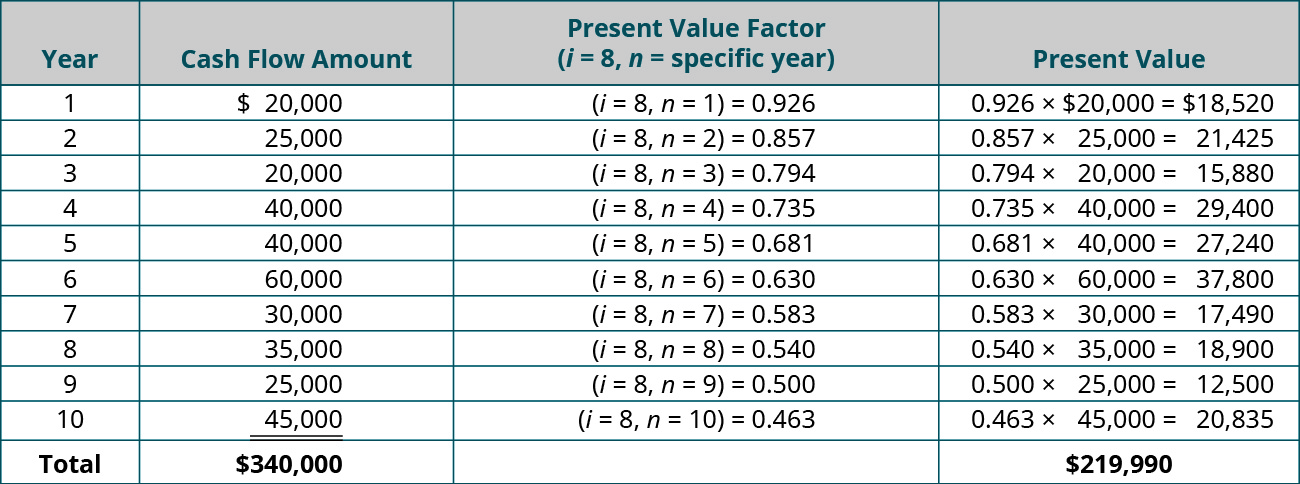The Present Value of ?1 table is used because, each year, a new “lump sum” cash flow is received, so the cash flow in each period is different. The cash flows are treated as one-time lump sum payouts during that year. The present value for each period looks at each year’s present value factor at an interest rate of 8%. All the PVs are added together for a total present value of ?219,990. The initial investment of ?200,000 is subtracted from the ?219,990 to arrive at a positive NPV of ?19,990. In this case, the company would consider investment since the outcome is positive. (More complex considerations, such as depreciation, the effects of income taxes, and inflation, which could affect the overall NPV, are covered in advanced accounting courses.)

Analyzing a Postage Meter Investment

Yellow Industries is considering investment in a new postage meter system. The postage meter system would have an initial investment cost of ?135,000. Annual net cash flows are ?40,000 for the next 5 years, and the expected interest rate return is 10%. Calculate net present value and decide whether or not Yellow Industries should invest in the new postage meter system.

Solution

Use the Present Value of an Ordinary Annuity table. Present value factor at n = 5 and i = 10% is 3.791. Present value = 3.791 × ?40,000 = ?151,640. NPV = ?151,640 − ?135,000 = ?16,640. In this case, Yellow Industries should invest since the NPV is positive.

#### Calculation and Discussion of the Results of the Net Present Value Model

To demonstrate NPV, assume that a company, Rayford Machining, is considering buying a drill press that will have an initial investment cost of ?50,000 and annual cash flows of ?10,000 for the next 7 years. Assume that Rayford expects a 5% rate of return on such an investment. We need to determine the NPV when cash flows are equal. The present value factor (i = 5, n = 7) is 5.786 using the Present Value of an Ordinary Annuity table. We multiply 5.786 by the equal cash flow of ?10,000 to get a present value of ?57,860. NPV is found by taking the present value of ?57,860 and subtracting the initial investment of ?50,000 to arrive at ?7,860. This is a positive NPV, so the company would consider the investment.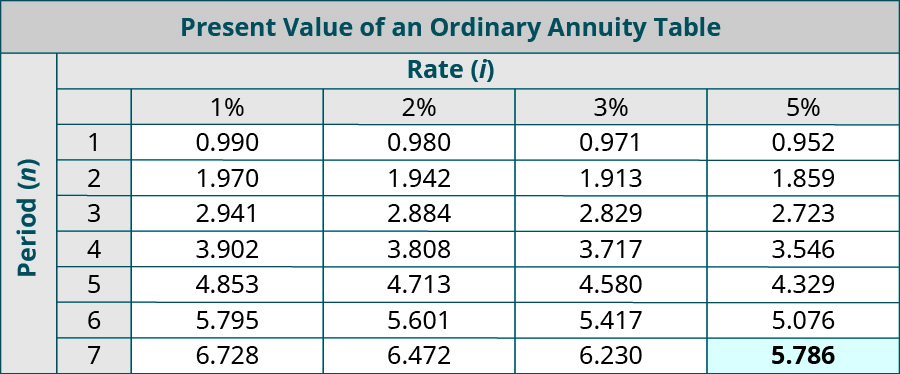Let’s say Rayford Machining has another option, Option B, for a drill press purchase with an initial investment cost of ?56,000 that produces present value cash flows of ?60,500. The profitability index is computed as follows.

$$\begin{array}{}\\ \\ \text{Option A:}\phantom{\rule{0.2em}{0ex}}\frac{?57,860}{?50,000}=1.157\hfill \\ \text{Option B:}\phantom{\rule{0.2em}{0ex}}\frac{?60,500}{?56,000}=1.080\hfill \end{array}$$

Based on this outcome, the company would invest in Option A, the project with a higher profitability potential of 1.157.

Now let’s assume cash flows are unequal. Unequal cash flow information for Rayford Machining is summarized here.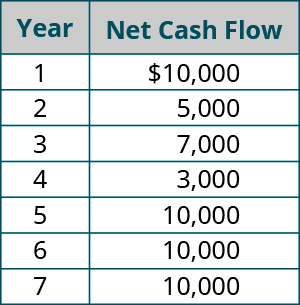To find the overall present value, the following calculations take place using the Present Value of ?1 table.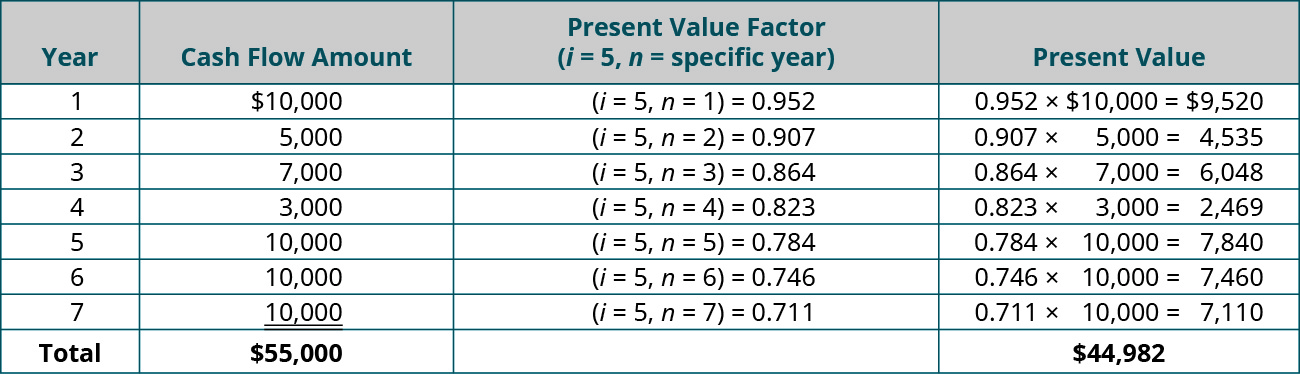The present value for each period looks at each year’s present value factor at an interest rate of 5%. All individual year present values are added together for a total present value of ?44,982. The initial investment of ?50,000 is subtracted from the ?44,982 to arrive at a negative NPV of ?5,018. In this case, Rayford Machining would not invest, since the outcome is negative. The negative NPV value does not mean the investment would be unprofitable; rather, it means the investment does not return the desired 5% the company is looking for in the investments that it makes.

#### Basic Characteristics of the Internal Rate of Return Model

The internal rate of return model allows for the comparison of profitability or growth potential among alternatives. All external factors, such as inflation, are removed from calculation, and the project with the highest return rate percentage is considered for investment.

IRR is the discounted rate (interest rate) point at which NPV equals zero. In other words, the IRR is the point at which the present value cash inflows equal the initial investment cost. To consider investment, IRR needs to meet or exceed the required rate of return for the investment type. If IRR does not meet the required rate of return, the company will forgo investment.

To find IRR using the present value tables, we need to know the cash flow number of return periods (n) and the intersecting present value factor. To calculate present value factor, we use the following formula.We find the present value factor in the present value table in the row with the corresponding number of periods (n). We find the matching interest rate (i) at this present value factor. The corresponding interest rate at the number of periods (n) is the IRR. When cash flows are equal, use the Present Value of an Ordinary Annuity table to find IRR.

For example, a car manufacturer needs to replace welding equipment. The initial investment cost is ?312,000 and each annual net cash flow is ?49,944 for the next 9 years. We need to find the internal rate of return for this welding equipment. The expected rate of return for such a purchase is 6%. In this case, n = 9 and the present value factor is computed as follows.

$$\text{Present Value Factor}=\frac{?312,000}{?49,944}=6.247\phantom{\rule{0.2em}{0ex}}\text{(rounded)}$$

Looking at the Present Value of an Ordinary Annuity table, where n = 9 and the present value factor is 6.247, we discover that the corresponding return rate is 8%. This exceeds the expected return rate, so the company would typically invest in the project.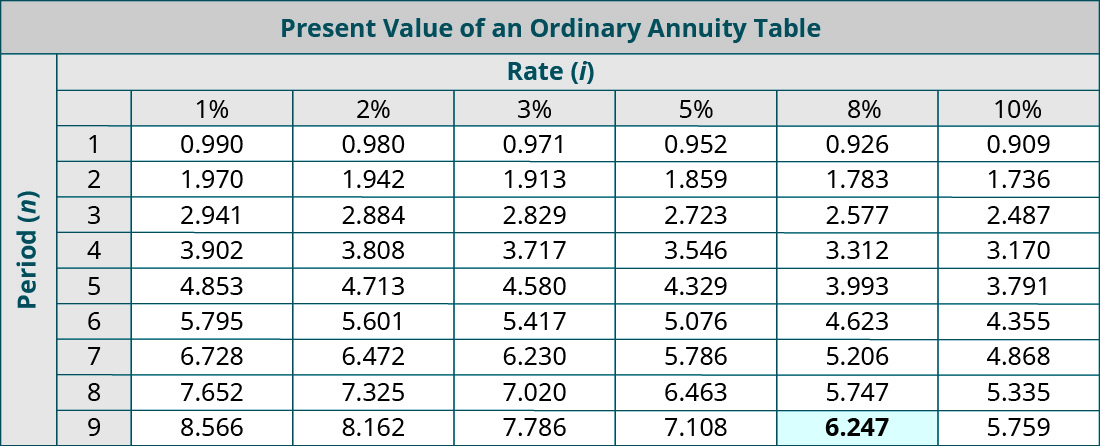If there is more than one viable option, the company will select the alternative with the highest IRR that exceeds the expected rate of return.

Our tables are limited in scope, and therefore, a present value factor may fall in between two interest rates. When this is the case, you may choose to identify an IRR range instead of a single interest rate figure. A spreadsheet program or financial calculator can produce a more accurate result and can also be used when cash flows are unequal.

#### Calculation and Discussion of the Results of the Internal Rate of Return Model

Assume that Rayford Machining wants to know the internal rate of return for the new drill press. The drill press has an initial investment cost of ?50,000 and an annual cash flow of ?10,000 for each of the next seven years. The company expects a 7% rate of return on this type of investment. We calculate the present value factor as:

$$\text{Present Value Factor}=\frac{?50,000}{?10,000}=5.000$$

Scanning the Present Value of an Ordinary Annuity table reveals that the interest rate where the present value factor is 5 and the number of periods is 7 is between 8 and 10%. Since the required rate of return was 7%, Rayford would consider investment in this metal press machine.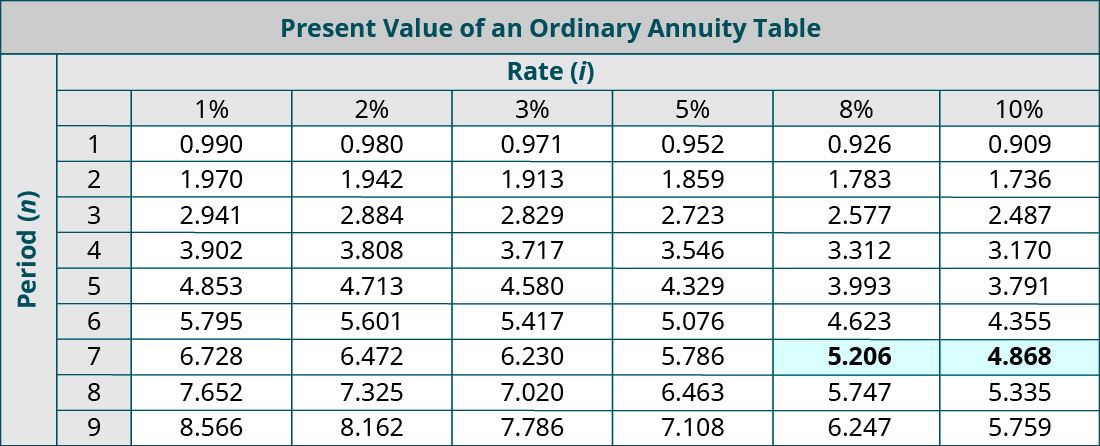Consider another example using Rayford, where they have two drill press purchase options. Option A has an IRR between 8% and 10%. The other option, Option B, has an initial investment cost of ?60,500 and equal annual net cash flows of ?13,256 for the next seven years. We calculate the present value factor as:

$$\text{Present Value Factor}=\frac{?60,500}{?13,256}=4.564\phantom{\rule{0.2em}{0ex}}\text{(rounded)}$$

Scanning the Present Value of an Ordinary Annuity table reveals that, when the present value factor is 4.564 and the number of periods is 7, the interest rate is 12%. This not only exceeds the 7% required rate, it also exceeds Option A’s return of 8% to 10%. Therefore, if resources were limited, Rayford would select Option B over Option A.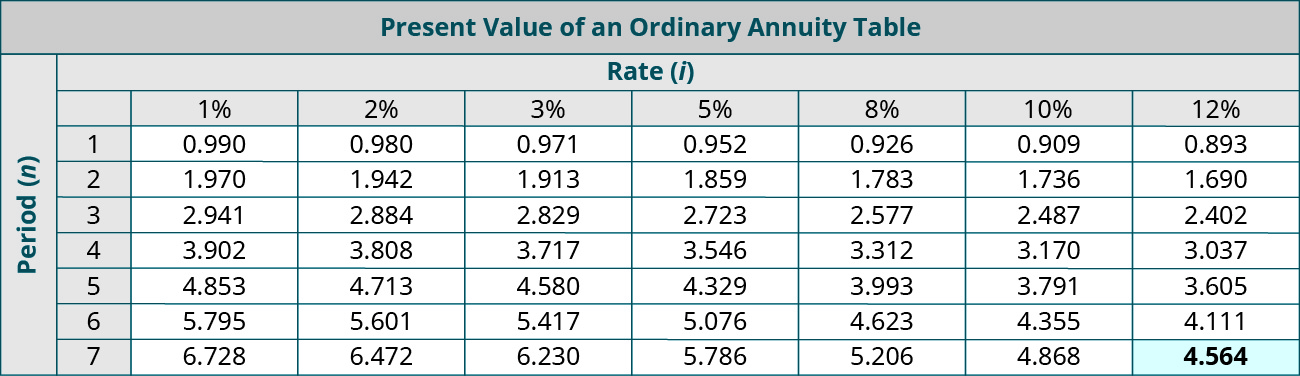### Final Summary of the Discounted Cash Flow Models

The internal rate of return (IRR) and the net present value (NPV) methods are types of discounted cash flow analysis that require taking estimated future payments from a project and discounting them into present values. The difference between the two methods is that the NPV calculation determines the project’s estimated return in dollars and the IRR provides the percentage rate of return from a project needed to break even.

When the NPV is determined to be ?0, the present value of the cash inflows and the present value of the cash outflows are equal. For example, assume that the present value of the cash inflows is ?10,000 and the present value of the cash outflows is also ?10,000. In this example, the NPV would be ?0. At a net present value of zero, the IRR would be exactly equal to the interest rate that was used to perform the NPV calculation. For example, in the previous example, where both the cash inflows and the cash outflows have present values of ?10,000 and the NPV is ?0, assume that they were discounted at an 8% interest rate. If you were to then calculate the internal rate of return, the IRR would be 8%, the same interest rate that gave us an NPV of ?0.

Overall, it is important to understand that a company must consider the time value of money when making capital investment decisions. Knowing the present value of a future cash flow enables a company to better select between alternatives. The net present value compares the initial investment cost to the present value of future cash flows and requires a positive outcome before investment. The internal rate of return also considers the present value of future cash flows but considers profitability stated in terms of percentage of return on the investment or project. These models allows two or more options to be compared to eliminate bias with raw financial figures.

Choosing Investments

Companies are presented with viable alternatives that sometimes produce nearly identical results and profitability goals. If they have the ability to invest in both alternatives, they may do so. But what about when resources are constrained? How do they choose which investment is best for their company?

Consider this: you have two projects that met the payback period and accounting rate of return screenings identically. Project 1 produced an NPV of ?45,000 and had an IRR between 5% and 8%. Project 2 produced a NPV of ?35,000 and had an IRR of 10%. This leaves you with a difficult choice, since each alternative has a measurement that exceeds the other and the other variables are the same. Which project would you invest in and why?

### Key Concepts and Summary

• The discounted cash flow model assigns values to a project’s alternatives using time value of money and discounts future rates back to present value. Two measurement tools are used in discounted cash flows: net present value and internal rate of return.
• Net present value considers an expected rate of return, converts future cash flows into present value, and compares that to the initial investment cost. If the outcome is positive, the company would look to invest in the project.
• Internal rate of return shows the profitability of an investment, where NPV equals zero. If the corresponding interest rate exceeds the expected rate of return, the company would invest in the project.

(Figure)The NPV method assumes that cash inflows associated with a particular investment occur when?

1. only at the time of the initial investment
2. only at the end of the year
3. only at the beginning of the year
4. at any of these times

D

(Figure)Which of the following does not assign a value to a business opportunity using time-value measurement tools?

1. internal rate of return (IRR) method
2. net present value (NPV)
3. discounted cash flow model
4. payback period method

(Figure)Which of the following discounts future cash flows to their present value at the expected rate of return, and compares that to the initial investment?

1. internal rate of return (IRR) method
2. net present value (NPV)
3. discounted cash flow model
4. future value method

B

(Figure)This calculation determines profitability or growth potential of an investment, expressed as a percentage, at the point where NPV equals zero

1. internal rate of return (IRR) method
2. net present value (NPV)
3. discounted cash flow model
4. future value method

(Figure)What is the difference between the discount rate used for net present value and the internal rate of return methods?

For NPV computations, a minimum required rate of return or discount rate is used as a screening tool to determine whether or not a capital investment decision meets a predetermined set of criteria. If the net present value of an investment is positive, then the capital investment generates an actual return greater than the discount rate and the project will be deemed acceptable. The discount rate, however, is not the actual rate of return earned by the project.
The internal rate of return determines the actual rate of return that a project earns.

(Figure)Briefly explain how NPV is computed and interpreted.

(Figure)What is the basic benefit of using IRR?

The internal rate of return (IRR) shows the profitability or growth potential of an investment. All external factors are removed from calculation, such as inflation concerns, and the project with the highest return rate percentage is considered for investment. A company may have several viable alternatives that need a differentiating factor. IRR gives a solid differentiation, presented as a percentage rather than a dollar figure, as seen in NPV. This removes bias from projects with dissimilar NPVs and is a way to compare more than one option.

(Figure)How is the IRR determined if there are uneven cash flows?

(Figure)Project A costs ?5,000 and will generate annual after-tax net cash inflows of ?1,800 for five years. What is the NPV using 8% as the discount rate?

(Figure)Project B cost ?5,000 and will generate after-tax net cash inflows of ?500 in year one, ?1,200 in year two, ?2,000 in year three, ?2,500 in year four, and ?2,000 in year five. What is the NPV using 8% as the discount rate? For further instructions on net present value in Excel, see Appendix C.

(Figure)Gardner Denver Company is considering the purchase of a new piece of factory equipment that will cost ?420,000 and will generate ?95,000 per year for 5 years. Calculate the IRR for this piece of equipment. For further instructions on internal rate of return in Excel, see Appendix C.

(Figure)Consolidated Aluminum is considering the purchase of a new machine that will cost ?308,000 and provide the following cash flows over the next five years: ?88,000, 92,000, ?91,000, ?72,000, and ?71,000. Calculate the IRR for this piece of equipment. For further instructions on internal rate of return in Excel, see Appendix C.

(Figure)Redbird Company is considering a project with an initial investment of ?265,000 in new equipment that will yield annual net cash flows of ?45,800 each year over its seven-year life. The company’s minimum required rate of return is 8%. What is the internal rate of return? Should Redbird accept the project based on IRR?

(Figure)Project X costs ?10,000 and will generate annual net cash inflows of ?4,800 for five years. What is the NPV using 8% as the discount rate?

(Figure)Project Y cost ?8,000 and will generate net cash inflows of ?1,500 in year one, ?2,000 in year two, ?2,500 in year three, ?3,000 in year four and ?2,000 in year five. What is the NPV using 8% as the discount rate?

(Figure)Caduceus Company is considering the purchase of a new piece of factory equipment that will cost ?565,000 and will generate ?135,000 per year for 5 years. Calculate the IRR for this piece of equipment. For further instructions on internal rate of return in Excel, see Appendix C.

(Figure)Garnette Corp is considering the purchase of a new machine that will cost ?342,000 and provide the following cash flows over the next five years: ?99,000, ?88,000, ?92,000, ?87,000, and ?72,000. Calculate the IRR for this piece of equipment. For further instructions on internal rate of return in Excel, see Appendix C.

(Figure)Wallace Company is considering two projects. Their required rate of return is 10%.

Project A Project B
Initial investment ?170,000 ?48,000
Annual cash flows ?41,352 ?12,022
Life of the project 6 years 5 years

Which of the two projects, A or B, is better in terms of internal rate of return?

(Figure)Falkland, Inc., is considering the purchase of a patent that has a cost of ?50,000 and an estimated revenue producing life of 4 years. Falkland has a cost of capital of 8%. The patent is expected to generate the following amounts of annual income and cash flows: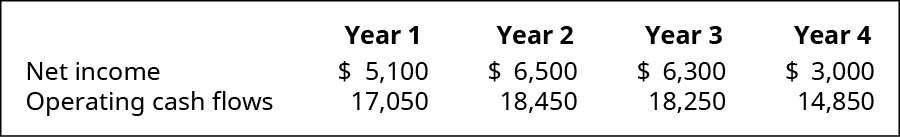1. What is the NPV of the investment?
2. What happens if the required rate of return increases?

(Figure)There are two projects under consideration by the Rainbow factory. Each of the projects will require an initial investment of ?35,000 and is expected to generate the following cash flows: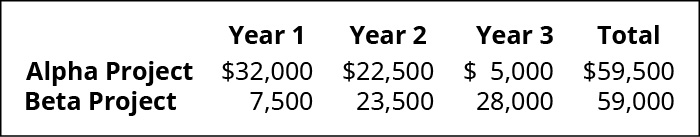If the discount rate is 12%, compute the NPV of each project.

(Figure)There are two projects under consideration by the Rainbow factory. Each of the projects will require an initial investment of ?35,000 and is expected to generate the following cash flows: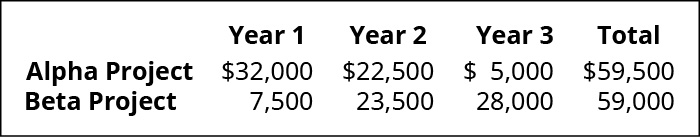Use the information from the previous exercise to calculate the internal rate of return on both projects and make a recommendation on which one to accept. For further instructions on internal rate of return in Excel, see Appendix C.

(Figure)Pompeii’s Pizza has a delivery car that it uses for pizza deliveries. The transmission needs to be replaced and there are several other repairs that need to be done. The car is nearing the end of its life, so the options are to either overhaul the car or replace it with a new car. Pompeii’s has put together the following budgetary items: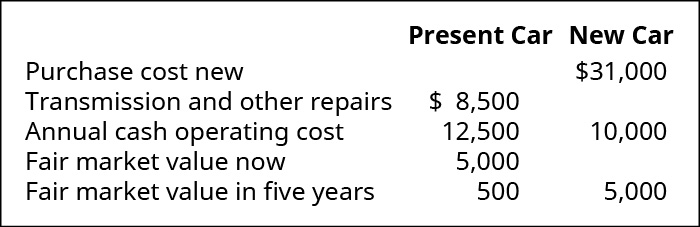If Pompeii’s replaces the transmission of the pizza delivery vehicle, they expect to be able to use the vehicle for another 5 years. If they sell the old vehicle and purchase a new vehicle, they will use that vehicle for 5 years and then trade it in for another new pizza delivery vehicle. If they trade for the new delivery vehicle, their operating expenses will decrease because the new vehicle is more gas efficient and the maintenance on a new car is less. This project is analyzed using a discount rate of 12%. What should Pompeii’s do?

(Figure)Pitt Company is considering two alternative investments. The company requires a 12% return from its investments. Neither option has a salvage value.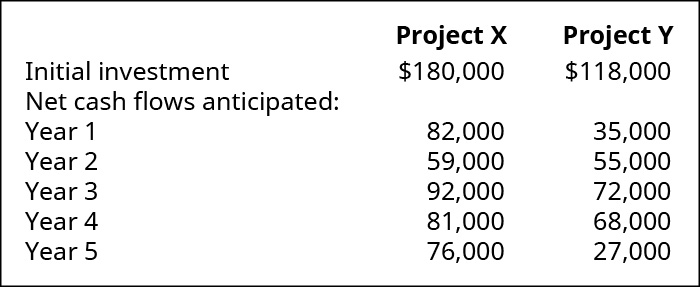Compute the IRR for both projects and recommend one of them. For further instructions on internal rate of return in Excel, see Appendix C.

(Figure)Mason, Inc., is considering the purchase of a patent that has a cost of ?85,000 and an estimated revenue producing life of 4 years. Mason has a required rate of return that is 12% and a cost of capital of 11%. The patent is expected to generate the following amounts of annual income and cash flows: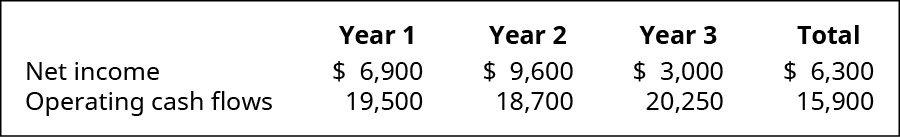1. What is the NPV of the investment?
2. What happens if the required rate of return increases?

(Figure)There are two projects under consideration by the Rainbow factory. Each of the projects will require an initial investment or ?28,000 and is expected to generate the following cash flows: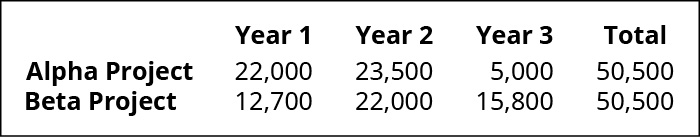If the discount rate is 5% compute the NPV of each project and make a recommendation of the project to be chosen.

(Figure)Use the information from the previous exercise to calculate the Internal Rate of Return on both projects and make a recommendation regarding which one to accept.

(Figure)D&M Pizza has a delivery car that is uses for pizza deliveries. The transmission needs to be replaced, and there are several other repairs that need to be done. The car is nearing the end of its life, so the options are to either overhaul the car or replace it with a new car. D&M’s has put together the following budgetary items: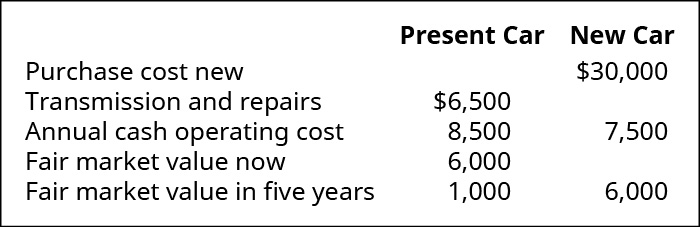If D&M replaces the transmission of the pizza delivery vehicle, they expect to be able to use the vehicle for another 5 years. If they purchase a new vehicle, they will sell the existing one and use the new vehicle for 5 years and then trade it in for another new pizza delivery vehicle. If they trade for the new delivery vehicle, their operating expenses will decrease because the new vehicle is more gas efficient. This project is analyzed using a discount rate of 15%. What should D&M do?

(Figure)Joliet Company is considering two alternative investments. The company requires an 18% return from its investments.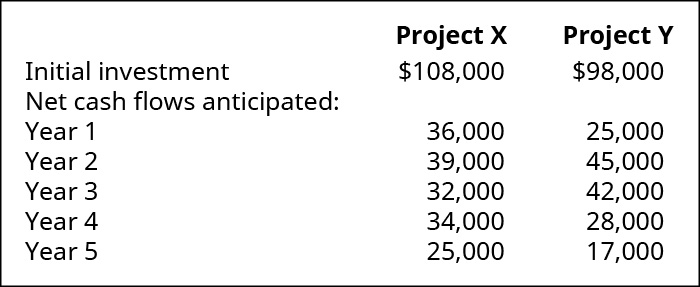Compute the IRR for both Projects and recommend one of them. For further instructions on internal rate of return in Excel, see Appendix C.

The following are independent situations. For each capital budgeting project, indicate whether management should accept or reject the project and list a brief reason why.

(Figure)Midas Corp. evaluated a potential investment and determined the NPV to be zero. Midas Corp.’s required rate of return is 9.1% and its cost of capital is 6.4%.

(Figure)Giorgio Co. is looking at an investment project with an internal rate of return of 10.8%. The initial outlay for the investment is ?90,000. The hurdle rate or minimum acceptable rate of return is 10.2%.

(Figure)Dinaro Inc. is looking at an investment project that has an NPV of (?5,000). The hurdle rate is 8%.

(Figure)You begin a new job at Cabrera Medical Supplies. The company is considering a new accounting system, with an initial investment of about half a million dollars for new software and hardware. You are excited for the opportunity to apply your managerial accounting skills regarding screening and preference methods to decide on the best system for the company. Your boss is a little old-school, and when you mention some of the things you learned in managerial accounting, he says, “Discounted cash flow methods are not the only way to approach this. I have more of a gut reaction approach that blows most managers out of the water when they become absorbed by discounted cash flow methods (DCF).”

How would you react and what would you discuss with your boss?

### Glossary

discounted cash flow model
assigns a value to a business opportunity using time-value measurement tools
internal rate of return method (IRR)
calculation to determine profitability or growth potential of an investment, expressed as a percentage, at the point where NPV equals zero
net present value method (NPV)
discounts future cash flows to their present value at the expected rate of return, and compares that to the initial investment